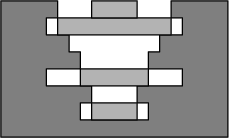Your browser (Unknown 0) is no longer supported. Some parts of the website may not work correctly. Please update your browser.

#### FallingDisks

Determine the number of disks that fit into the well.

There is an old dry well. Its sides are made of concrete rings. Each such ring is one meter high, but the rings can have different (internal) diameters. Nevertheless, all the rings are centered on one another. The well is N meters deep; that is, there are N concrete rings inside it.

You are about to drop M concrete disks into the well. Each disk is one meter thick, and different disks can have different diameters. Once each disk is dropped, it falls down until:

• it hits the bottom of the well;
• it hits a ring whose internal diameter is smaller then the disk's diameter; or
• it hits a previously dropped disk.

(Note that if the internal diameter of a ring and the diameter of a disk are equal, then the disk can fall through the ring.)

The disks you are about to drop are ready and you know their diameters, as well as the diameters of all the rings in the well. The question arises: how many of the disks will fit into the well?

Write a function:

class Solution { public int solution(int[] A, int[] B); }

that, given two arrays of integers − A, containing the internal diameters of the N rings (in top-down order), and B, containing the diameters of the M disks (in the order they are to be dropped) − returns the number of disks that will fit into the well.

For example, given the following two arrays:

A = 5 B = 2 A = 6 B = 3 A = 4 B = 5 A = 3 B = 2 A = 6 B = 4 A = 2 A = 3

the function should return 4, as all but the last of the disks will fit into the well. The figure shows the situation after dropping four disks.Write an efficient algorithm for the following assumptions:

• N and M are integers within the range [1..200,000];
• each element of arrays A and B is an integer within the range [1..1,000,000,000].